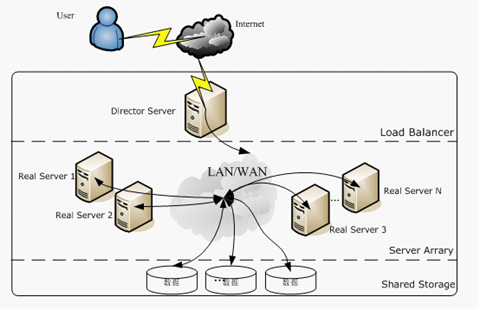# 聚类算法评估指标

• 高的类内 (intra-cluster) 相似度
• 低的类间 (inter-cluster) 相似度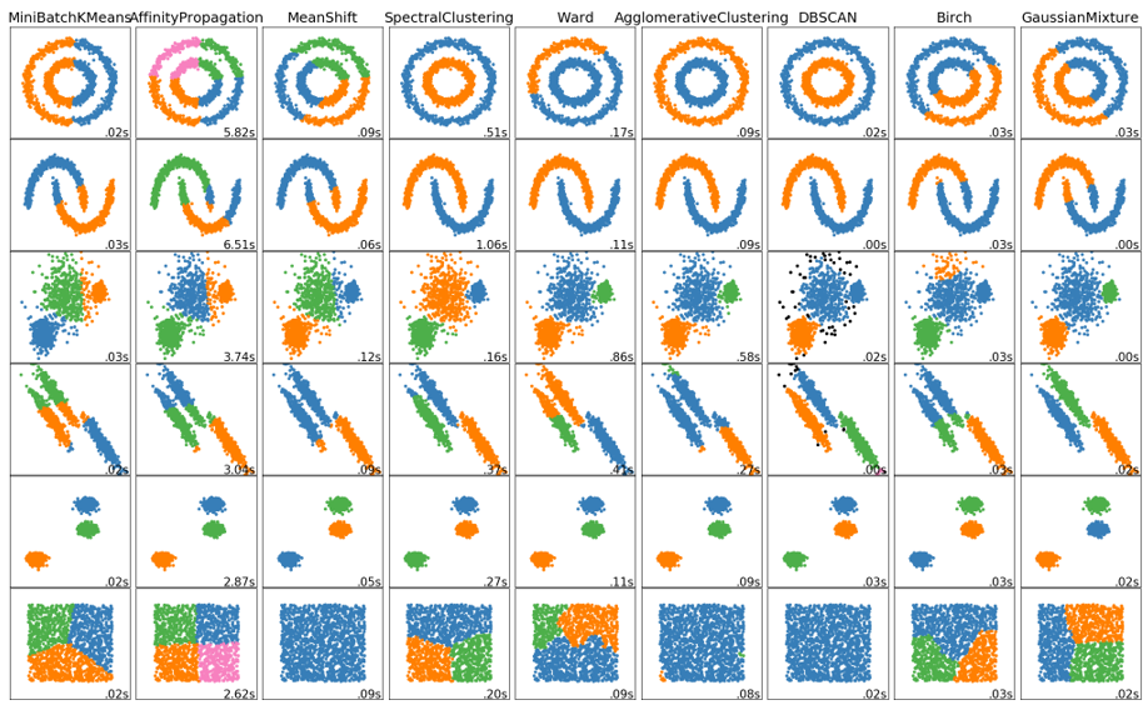• 内部评估的方法：通过一个单一的量化得分来评估算法好坏；该类型的方法
• 外部评估的方法：通过将聚类结果与已经有“ground truth”分类进行对比。要么通过人类进行手动评估，要么通过一些指标在特定的应用场景中进行聚类用法的评估。不过该方法是有问题的，如果真的有了label，那么还需要聚类干嘛，而且实际应用中，往往都没label；另一方面，这些label只反映了数据集的一个可能的划分方法，它并不能告诉你存在一个不同的更好的聚类算法。

## 内部评价指标

• 那些高分的算法不一定可以适用于高效的信息检索应用场景；
• 这些评估方法对某些算法有倾向性，如k-means聚类都是基于点之间的距离进行优化的，而那些基于距离的内部评估方法就会过度的赞誉这些生成的聚类结果。

### SSE(和方差)

$$\sum_{i=1}^{n}{(y_i – \hat{y_i})^2}$$

SSE越接近于0，说明模型选择和拟合更好，数据预测也越成功。

#断崖图选取最优K值
import pandas as pd
from sklearn.cluster import KMeans
import matplotlib.pyplot as plt
# '利用SSE选择k'
SSE = []  # 存放每次结果的误差平方和
for k in range(1,9):
estimator = KMeans(n_clusters=k)  # 构造聚类器
estimator.fit(df[['calories','sodium','alcohol','cost']])
SSE.append(estimator.inertia_)
N = range(1,9)
plt.xlabel('k')
plt.ylabel('SSE')
plt.plot(N,SSE,'o-')
plt.show()


### 轮廓系数 Silhouette Coefficient

$$s = \frac{b-a}{\max(a,b)}$$

from sklearn import metrics
from sklearn.metrics import pairwise_distances
from sklearn import datasets
X = dataset.data
y = dataset.target

import numpy as np
from sklearn.cluster import KMeans
kmeans_model = KMeans(n_clusters=3, random_state=1).fit(X)
labels = kmeans_model.labels_
metrics.silhouette_score(X, labels, metric='euclidean')


### Calinski-Harabaz Index

$$s(k)=\frac{tr(B_k)}{tr(W_k)}\frac{m-k}{k-1}$$

• 当 cluster （簇）密集且分离较好时，分数更高，这与一个标准的 cluster（簇）有关。
• 得分计算很快与轮廓系数的对比，最大的优势：快！相差几百倍！毫秒级。

• 凸的簇的 Calinski-Harabaz index（Calinski-Harabaz 指数）通常高于其他类型的 cluster（簇），例如通过 DBSCAN 获得的基于密度的 cluster（簇）。所以不适合基于密度的聚类算法，DBSCAN。
import numpy as np
from sklearn.cluster import KMeans

kmeans_model = KMeans(n_clusters=3, random_state=1).fit(X)
labels = kmeans_model.labels_
print(metrics.calinski_harabaz_score(X, labels))


### Compactness(紧密性)(CP)

CP计算每一个类各点到聚类中心的平均距离CP越低意味着类内聚类距离越近。著名的 K-Means 聚类算法就是基于此思想提出的。缺点：没有考虑类间效果。

$$\overline{CP}_i = \frac{1}{n_i} \sum_{x\in C_i} \mid\mid x_i – \mu_i \mid\mid$$

$$\overline{CP} =\frac{1}{k} \sum_{k=1}^k \overline{CP}_k$$

### Separation(间隔性)(SP)

SP计算各聚类中心两两之间平均距离，SP越高意味类间聚类距离越远。缺点：没有考虑类内效果。

$$\overline{SP} = \frac{2}{k_2 – k} \sum_{i=1}^k \sum_{j=i+1}^k \mid\mid \mu_i – \mu_j \mid\mid$$

### Davies-Bouldin Index(戴维森堡丁指数)(分类适确性指标)(DB)(DBI)

DB计算任意两类别的类内距离平均距离(CP)之和除以两聚类中心距离求最大值。DB越小意味着类内距离越小同时类间距离越大。该指标的计算公式：

$$\text{DB}=\frac{1}{n} \sum_{i=1}^{n} \max _{j \neq i}(\frac{\sigma_{i}+\sigma_{j}}{d(c_{i}, c_{j})})$$

from sklearn import datasets
from sklearn.cluster import KMeans
from sklearn.metrics import davies_bouldin_score
from sklearn.datasets.samples_generator import make_blobs

X, y_true = make_blobs(n_samples=300, centers=4,
cluster_std=0.50, random_state=0)

# K-Means
kmeans = KMeans(n_clusters=4, random_state=1).fit(X)

# we store the cluster labels
labels = kmeans.labels_

print(davies_bouldin_score(X, labels))


### Dunn Validity Index (邓恩指数)(DVI)

DVI计算任意两个簇元素的最短距离(类间)除以任意簇中的最大距离(类内)。DVI越大意味着类间距离越大同时类内距离越小。

$$D=\frac{\min _{1 \leq i<j \leq n} d(i, j)}{\max _{1 \leq k \leq n} d^{\prime}(k)}$$

• 类间距离$d(i,j)$可以是任意的距离测度，例如两个类别的中心点的距离；
• 类内距离$d'(k)$可以以不同的方法去测量，例如类别kk中任意两点之间距离的最大值。

import pandas as pd
from sklearn import datasets
from jqmcvi import base

df = pd.DataFrame(X.data)

# K-Means
from sklearn import cluster
k_means = cluster.KMeans(n_clusters=3)
k_means.fit(df) #K-means training
y_pred = k_means.predict(df)

# We store the K-means results in a dataframe
pred = pd.DataFrame(y_pred)
pred.columns = ['Type']

# we merge this dataframe with df
prediction = pd.concat([df, pred], axis = 1)

# We store the clusters
clus0 = prediction.loc[prediction.Species == 0]
clus1 = prediction.loc[prediction.Species == 1]
clus2 = prediction.loc[prediction.Species == 2]
cluster_list = [clus0.values, clus1.values, clus2.values]

print(base.dunn(cluster_list))


## 外部评价指标

### 纯度（Purity）

$$\text{purity}(\Omega, \mathbb{C})=\frac{1}{N} \sum_{k} \max _{j} |\omega_{k} \cap c_{j} |$$

• $\Omega = \{w_1,w_2,…,w_k\}$是聚类的集合，$w_k$表示第k个聚类的集合。
• $\mathbb{C} = \{c_1,c_2,…,c_j\}$是文档集合，$c_j$表示第J个文档。
• $N$表示文档总数。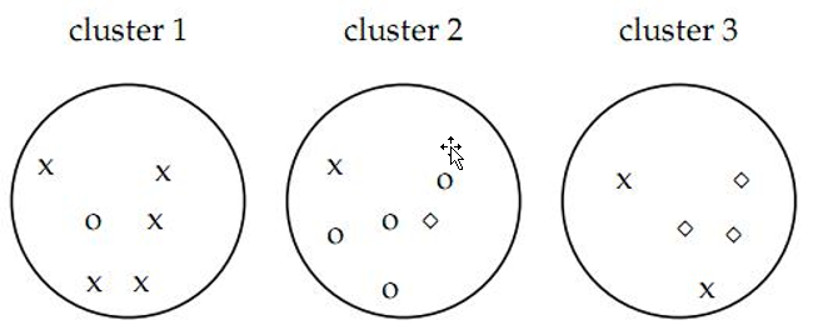def purity(result, label):
# 计算纯度

total_num = len(label)
cluster_counter = collections.Counter(result)
original_counter = collections.Counter(label)

t = []
for k in cluster_counter:
p_k = []
for j in original_counter:
count = 0
for i in range(len(result)):
if result[i] == k and label[i] == j: # 求交集
count += 1
p_k.append(count)
temp_t = max(p_k)
t.append(temp_t)

return sum(t)/total_num


### 标准化互信息（NMI）

$$\text{NMI}(\Omega, C)=\frac{I(\Omega ; C)}{(H(\Omega)+H(C) / 2)}$$

$$c =\sum_{k}\sum_{j}P(w_{k} \cap c_{j}) \log \frac{P(w_{k} \cap c_{j})}{P(w_{k}) P(c_{j})} =\sum_{k} \sum_{j} \frac{|w_{k} \cap c_{j}|}{N} \log \frac{N|w_{k} \cap c_{j}|}{|w_{k}t||c_{j}|}$$

$$H(\Omega )= -\sum_{k}P(w_k)\log P(w_k) = -\sum_{k}\frac{|w_k|}{N}\log\frac{|w_k|}{N}$$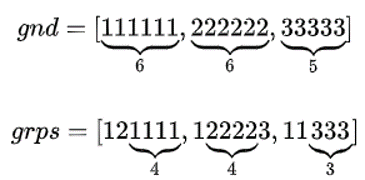gnd 是 ground truth 的意思，grps 表示聚类后的 groups. 问题：计算序列 gnd 和 grps 的 NMI.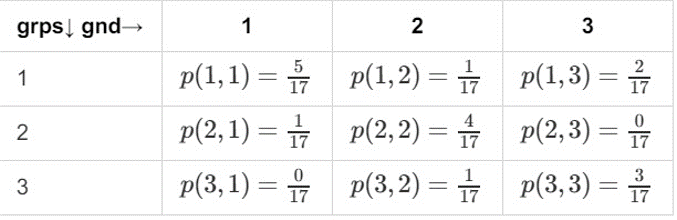$$P(gnd)=(\frac{6}{17},\frac{6}{17},\frac{5}{17})$$

$$P(grps)=(\frac{8}{17},\frac{5}{17},\frac{4}{17})$$

$$H(gnd) = 1.58$$

$$H(grps) = 1.522$$

$$H(gnd|grps) = 1.014$$

$$I(gnd;grps) = H(gnd)-H(gnd|grps)=0.564$$

$$\text{NMI} = \frac{2\times I(gnd;grps)}{H(gnd)+H(grps)} \approx 0.3649$$

def NMI(result, label):
# 标准化互信息

total_num = len(label)
cluster_counter = collections.Counter(result)
original_counter = collections.Counter(label)

# 计算互信息量
MI = 0
eps = 1.4e-45 # 取一个很小的值来避免log 0

for k in cluster_counter:
for j in original_counter:
count = 0
for i in range(len(result)):
if result[i] == k and label[i] == j:
count += 1
p_k = 1.0*cluster_counter[k] / total_num
p_j = 1.0*original_counter[j] / total_num
p_kj = 1.0*count / total_num
MI += p_kj * math.log(p_kj /(p_k * p_j) + eps, 2)

# 标准化互信息量
H_k = 0
for k in cluster_counter:
H_k -= (1.0*cluster_counter[k] / total_num) * math.log(1.0*cluster_counter[k] / total_num+eps, 2)
H_j = 0
for j in original_counter:
H_j -= (1.0*original_counter[j] / total_num) * math.log(1.0*original_counter[j] / total_num+eps, 2)

return 2.0 * MI / (H_k + H_j)


sklearn中自带的方法：

from sklearn.metrics.cluster import normalized_mutual_info_score
print(normalized_mutual_info_score([0, 0, 1, 1], [0, 0, 1, 1]))


$$H(U)=\sum_{i=1}^{|U|}P(i) \log (P(i))$$

$$H(V)=\sum_{j=1}^{|V|}{P}'(j) \log ({P}'(j))$$

• $P(i) = \frac{|U_i|}{N}$是从U中随机选取的对象到类$U_i$的概率
• ${P}'(j) = \frac{|V_j|}{N}$从V中随机选取的对象到类$V_j$的概率

U与V之间的互信息（MI）定义为：

$$\text{MI}(U,V) = \sum_{i=1}^{|U|}\sum_{j=1}^{|V|}P(i,j) \log (\frac{P(i,j)}{P(i){P}'(j)})$$

$$\text{AMI} = \frac{\text{MI}-E[\text{MI}]}{max(H(U),H(V)-E([\text{MI}]))}$$

MI的期望可以用以下公式来计算。在这个方程式中，$a_i=|U_i|$为$U_i$元素的数量，$b_j=|V_j|$为$V_j$元素的数量：

$$\mathbf{E}\{MI(U, V)\}=\sum_{i=1}^{R} \sum_{j=1}^{C} \sum_{n_{i j}=\max (a_{i}+b_{j}-N, 0)}^{\min (a_{i}, b_{j})} \frac{n_{ij}}{N} \log (\frac{N \cdot n_{ij}}{a_{i} b_{j}}) \frac{a_{i}!b_{j}!(N-a_{i})!(N-b_{j})!}{N!n_{i j} !(a_{i}-n_{i j})!(b_{j}-n_{i j})!(N-a_{i}-b_{j}+n_{i j})!}$$

• 随机（统一）标签分配的AMI评分接近0）
• 有界范围 [0, 1]: 接近 0 的值表示两个主要独立的标签分配，而接近 1 的值表示重要的一致性。此外，正好 0 的值表示 purely（纯粹） 独立标签分配，正好为 1 的 AMI 表示两个标签分配相等（有或者没有 permutation）。
• 对簇的结构没有作出任何假设: 可以用于比较聚类算法

• 与 inertia 相反， MI-based measures 需要了解 ground truth classes，而在实践中几乎不可用，或者需要人工标注或手动分配（如在监督学习环境中）。然而，基于 MI-based measures （基于 MI 的测量方式）也可用于纯无人监控的设置，作为可用于聚类模型选择的 Consensus Index （共识索引）的构建块。
• NMI 和 MI 没有调整机会。
from sklearn import metrics
labels_true = [0, 0, 0, 1, 1, 1]
labels_pred = [0, 0, 1, 1, 2, 2]



### Rand index兰德指数

Positive:

• TP 将两篇相似文档归入一个簇 (同 – 同)
• TN 将两篇不相似的文档归入不同的簇 (不同 – 不同)

Negative:

• FP 将两篇不相似的文档归入同一簇 (不同 – 同)
• FN 将两篇相似的文档归入不同簇 (同- 不同) (worse)

RI 则是计算「正确决策」的比率(精确率, accuracy)：

$$RI=\frac{TP+TN}{TP+FP+TF+FN}=\frac{TP+TN}{C_N^2}$$

RI取值范围为[0,1]，值越大意味着聚类结果与真实情况越吻合。

def contingency_table(result, label):

total_num = len(label)

TP = TN = FP = FN = 0
for i in range(total_num):
for j in range(i + 1, total_num):
if label[i] == label[j] and result[i] == result[j]:
TP += 1
elif label[i] != label[j] and result[i] != result[j]:
TN += 1
elif label[i] != label[j] and result[i] == result[j]:
FP += 1
elif label[i] == label[j] and result[i] != result[j]:
FN += 1
return (TP, TN, FP, FN)

def rand_index(result, label):
TP, TN, FP, FN = contingency_table(result, label)
return 1.0*(TP + TN)/(TP + FP + FN + TN)


$$\mathrm{ARI}=\frac{\mathrm{RI}-E[\mathrm{RI}]}{\max (\mathrm{RI})-E[\mathrm{RI}]}$$

ARI取值范围为[-1,1]，值越大意味着聚类结果与真实情况越吻合。从广义的角度来讲，ARI衡量的是两个数据分布的吻合程度。

• 对任意数量的聚类中心和样本数，随机聚类的ARI都非常接近于0
• 取值在［－1，1］之间，负数代表结果不好，越接近于1越好
• 对簇的结构不需作出任何假设：可以用于比较聚类算法。

• 与 inertia 相反，ARI 需要 ground truth classes 的相关知识，ARI需要真实标签，而在实践中几乎不可用，或者需要人工标注者手动分配（如在监督学习环境中）。然而，ARI 还可以在 purely unsupervised setting （纯粹无监督的设置中）作为可用于 聚类模型选择（TODO）的共识索引的构建块。
from sklearn import metrics
labels_true = [0, 0, 0, 1, 1, 1]
labels_pred = [0, 0, 1, 1, 2, 2]



### F值方法

$$Precision = P = \frac{TP}{TP+FP}$$

$$Recall = R = \frac{TP}{TP+FN}$$

$$F_{\beta}=\frac{(1+{\beta}^2)P\times R}{{\beta}^2P+R}$$

RI方法有个特点就是把准确率和召回率看得同等重要，事实上有时候我们可能需要某一特性更多一点，这时候就适合F值方法。

def precision(result, label):
TP, TN, FP, FN = contingency_table(result, label)
return 1.0*TP/(TP + FP)

def recall(result, label):
TP, TN, FP, FN = contingency_table(result, label)
return 1.0*TP/(TP + FN)

def F_measure(result, label, beta=1):
prec = precision(result, label)
r = recall(result, label)
return (beta*beta + 1) * prec * r/(beta*beta * prec + r)


### Fowlkes-Mallows scores

Fowlkes-Mallows Scores（FMI） FMI是成对的precision（精度）和recall（召回）的几何平均数。取值范围为 [0,1]，越接近1越好。定义为：

$$\text{FMI} = \frac{TP}{\sqrt{(TP+FP)(TP+FN)}}$$

from sklearn import metrics
labels_true = [0, 0, 0, 1, 1, 1]
labels_pred = [0, 0, 1, 1, 2, 2]

print(metrics.fowlkes_mallows_score(labels_true, labels_pred))


### 调和平均V-measure

• 同质性（homogeneity）：每个群集只包含单个类的成员。
• 完整性（completeness）：给定类的所有成员都分配给同一个群集。

$$h = 1- \frac{H(C|K)}{H(C)}$$

$$c = 1- \frac{H(K|C)}{H(K)}$$

$$H(C|K)= -\sum_{c=1}^{|C|}\sum_{k=1}^{|K|} \frac{n_{c,k}}{n} \cdot \log (\frac{n_{c,k}}{n})$$

$H(C)$是类熵，公式为：

$$H(C)= -\sum_{c=1}^{|C|} \frac{n_{c}}{n} \cdot \log (\frac{n_{c}}{n})$$

V-measure是同质性homogeneity和完整性completeness的调和平均数，V-measure取值范围为 [0,1]，越大越好，但当样本量较小或聚类数据较多的情况，推荐使用AMI和ARI。公式：

$$v = 2\cdot \frac{h\cdot c}{h+c}$$

from sklearn import metrics

labels_true = [0, 0, 0, 1, 1, 1]
labels_pred = [0, 0, 1, 1, 2, 2]

print(metrics.homogeneity_score(labels_true, labels_pred))
print(metrics.completeness_score(labels_true, labels_pred))
print(metrics.v_measure_score(labels_true, labels_pred))

• 分数明确：从0到1反应出最差到最优的表现；
• 解释直观：差的调和平均数可以在同质性和完整性方面做定性的分析；
• 对簇结构不作假设：可以比较两种聚类算法如k均值算法和谱聚类算法的结果。

• 以前引入的度量在随机标记方面没有规范化，这意味着，根据样本数，集群和先验知识，完全随机标签并不总是产生相同的完整性和均匀性的值，所得调和平均值V-measure也不相同。特别是，随机标记不会产生零分，特别是当簇的数量很大时。
• 当样本数大于一千，聚类数小于10时，可以安全地忽略该问题。对于较小的样本量或更大数量的集群，使用经过调整的指数（如调整兰德指数）更为安全。
• 这些指标要求的先验知识，在实践中几乎不可用或需要手动分配的人作注解者（如在监督学习环境中）。

### Jaccard 指数

$$J(A,B) = \frac{|A\cap B|}{|A\cup B|}= \frac{TP}{TP+FP+FN}$$

### Dice 指数

$$J(A,B) = \frac{|A\cap B|}{|A\cup B|}= \frac{2TP}{2TP+FP+FN}$$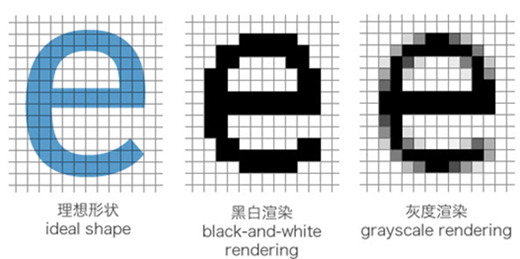##### 网站与APP开发中的字体设置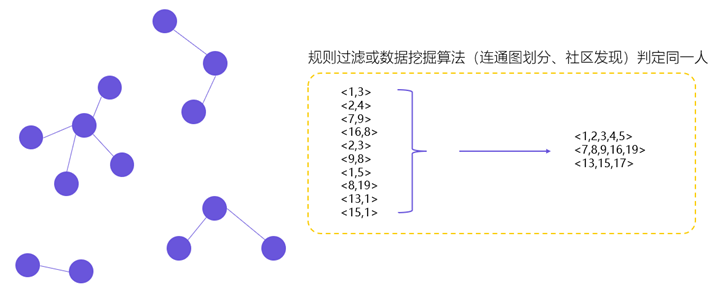##### 用户体系搭建之ID-Mapping